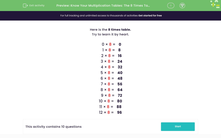# Know Your Multiplication Tables: The 8 Times Table

In this worksheet, students must recall multiplication facts from the 8 times table.Key stage:  KS 2

Curriculum topic:   Number: Multiplication and Division

Curriculum subtopic:   Use Multiplication/Division Facts (3, 4 and 8)

Popular topics:   Times Table worksheets

Difficulty level:#### Worksheet Overview

In this activity, we will be multiplying numbers by 8.

Here is the 8 times table.

Try to learn it by heart.

0 × 8 =   0

1 × 8 =   8

2 × 8 =   16

3 × 8 =   24

4 × 8 =   32

5 × 8 =   40

6 × 8 =   48

7 × 8 =   56

8 × 8 =   64

9 × 8 =   72

10 × 8 =   80

11 × 8 =   88

12 × 8 =   96

Numbers in the 8 times table end in 8, 6, 4, 2, or 0.

If you look at the facts above, you will spot that these numbers repeat in the one's place of each multiple:

8, 16, 24, 32, 40, 48, 56, 64, 72, 80, 88, 96

A tip for multiplying by eight is to double the number, then double the result and then double once more!

Let's look at an example

Example

Find 4 × 8.

We can double 4, then double the result, then double the next result too.

Double 4

4 × 2 = 8

Double 8

8 × 2 = 16

Double 16

16 × 2 = 31

4 × 8 = 32

Now you're ready to try some questions. Good luck!

### What is EdPlace?

We're your National Curriculum aligned online education content provider helping each child succeed in English, maths and science from year 1 to GCSE. With an EdPlace account you’ll be able to track and measure progress, helping each child achieve their best. We build confidence and attainment by personalising each child’s learning at a level that suits them.

Get started